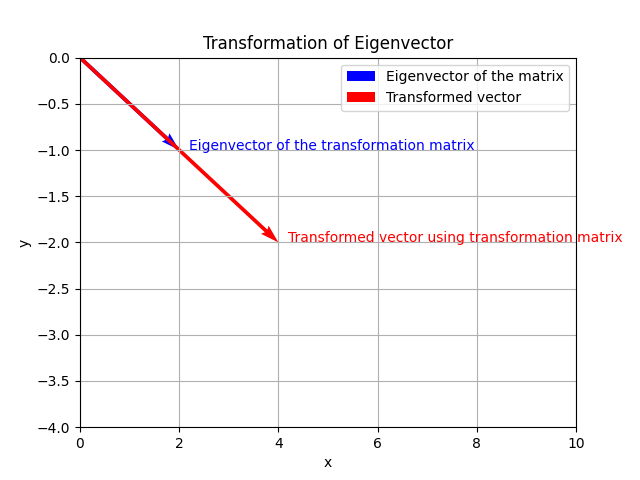Course Content

Mathematics for Data Analysis and Modeling

##Eigenvalues and Eigenvectors

Eigenvectors and eigenvalues are concepts related to linear transformations and matrices. An eigenvector v is a non-zero vector that results in a scaled version of itself when multiplied by a given matrix. The eigenvalue λ associated with an eigenvector represents the scalar value by which the eigenvector is scaled.If we have some matrix `A` and provide linear transformation `A * v`, where `v`- eigenvector of matrix `A`, we will get the vector with the same direction but with different length:## Calculating eigenvalues and eigenvectors

To find eigenvectors and corresponding eigenvalues of a matrix, we can use `np.linalg.eig()` method:In this example, we create a 3x3 `matrix` matrix. We then use the `np.linalg.eig()` method from `NumPy` to calculate the eigenvalues and eigenvectors. The function returns two arrays: eigenvalues contain the eigenvalues, and eigenvectors contain the corresponding eigenvectors.

## Practical applications

Eigenvalues ​​and vectors are often used to solve various applied problems. One of these problems is the problem of dimensionality reduction for which the PCA algorithm is used: this algorithm is based on using eigenvalues ​​of the feature covariance matrix.

Note

Dimensionality reduction is a fundamental problem in data analysis and machine learning, aiming to reduce the number of features or variables in a dataset while preserving as much relevant information as possible.